# 802.11be Transmitter Measurements

This example demonstrates how to measure transmitter modulation accuracy, spectral mask, and spectral flatness for IEEE® 802.11be™ waveforms.

### Introduction

In this example, you generate an oversampled IEEE 802.11be extremely high throughput (EHT) multi-user (MU) waveform, as defined in IEEE P802.11be/D2.0 . You can configure the example to generate orthogonal frequency-division multiple-access (OFDMA) waveforms, which transmit data for multiple users over different parts of the band, or non-OFDMA waveforms, which transmit data for a single user over the whole band. You can also introduce in-band distortion and spectral regrowth by using a high-power amplifier (HPA) model. You then perform the transmitter modulation accuracy, required spectral mask, and required spectral flatness measurement on the waveform for the measurement configuration specified in Section 36.3.19 of .

For each user, the example decodes the EHT Data field and measures the error vector magnitude (EVM) to determine the modulation accuracy after downsampling the waveform to baseband sampling rate. Additionally, for the non-OFDMA PPDU type, the example measures the spectral flatness of the recovered waveform. This diagram shows the example workflow.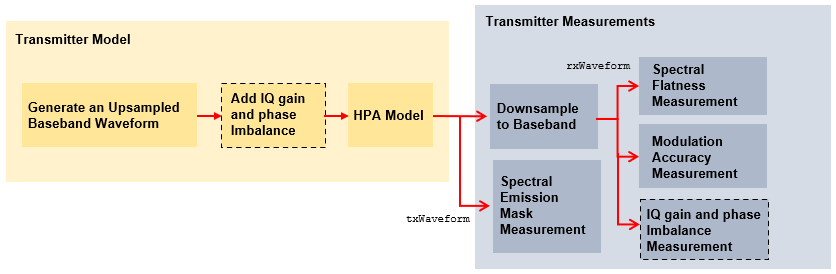### Simulation Setup

Configure the example to generate two EHT MU packets with a 10 microsecond idle period between each packet.

```numPackets =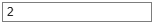2; idleTime =10;```

### 802.11be Waveform Configuration and Generation

The draft standard defines the EHT MU format for the transmission of non-OFDMA and OFDMA PPDU types. This example supports generation of non-OFDMA and OFDMA EHT MU PPDU types. For more information about the parameterization and generation of IEEE 802.11be EHT MU waveforms, see the 802.11be Waveform Generation example.

```savedState = rng(0); % Set random state ppduType ="OFDMA"; % Set ppduType to OFDMA or Non-OFDMA```

Configure transmission parameters of an EHT MU packet by using EHT MU configuration object `wlanEHTMUConfig` For an OFDMA PPDU type, create an OFDMA configuration for a 20 MHz EHT MU packet with allocation index 47 as defined in Table 36-34 of . This allocation has two 52-tone resource units (RUs) and one 106+26-tone multiple resource unit (MRU). This configuration specifies the transmission of a single user per RU. Set the transmission parameters for each user.

```if strcmp(ppduType,"OFDMA") allocationIndex = 47; % Allocation index 47 specifies three RUs and three users mcs = [12 5 4]; % Modulation and coding scheme per user spatialMapping = ["Direct" "Direct" "Direct"]; % Set spatial mapping property per RU apepLength = [1000 500 400]; % A-MPDU length pre-EOF padding in bytes per user numSTSs = [1 1 1]; % Number of space-time streams per user channelCoding = ["LDPC" "LDPC" "BCC"]; % Set channel coding property per user numTx = 1; % Number of transmit antennas cfgEHT = wlanEHTMUConfig(allocationIndex); chanBW = cfgEHT.ChannelBandwidth; numUsers = numel(cfgEHT.User); cfgEHT.NumTransmitAntennas = numTx; for i = 1:numUsers cfgEHT.RU{i}.SpatialMapping = spatialMapping(i); cfgEHT.User{i}.APEPLength = apepLength(i); cfgEHT.User{i}.MCS = mcs(i); cfgEHT.User{i}.NumSpaceTimeStreams = numSTSs(i); cfgEHT.User{i}.ChannelCoding = channelCoding(i); end end```

For the non-OFDMA PPDU type, create a full-band 320 MHz single-user (SU) MIMO configuration and set the transmission parameters of the user.

```if strcmp(ppduType,"Non-OFDMA") chanBW = "CBW320"; % Channel bandwidth mcs = 12; % Modulation and coding scheme apepLength = 8000; % A-MPDU length pre-EOF padding in bytes numTx = 1; % Number of transmit antennas cfgEHT = wlanEHTMUConfig(chanBW); % EHT MU configuration object numUsers = numel(cfgEHT.User); cfgEHT.NumTransmitAntennas = numTx; cfgEHT.RU{1}.SpatialMapping = "Direct"; cfgEHT.User{1}.APEPLength = apepLength; cfgEHT.User{1}.MCS = mcs; cfgEHT.User{1}.NumSpaceTimeStreams = numTx; cfgEHT.User{1}.ChannelCoding = "LDPC"; end```

To model the effect of an HPA on the waveform and view the out-of-band spectral emissions, the waveform must be oversampled. Generate the waveform using a larger IFFT than required for the nominal baseband rate, resulting in an oversampled waveform.

`osf =4; % Oversampling factor`

Create random bits for all packets.

```psduLen = psduLength(cfgEHT).*8; data = cell(1,numUsers); for i=1:numUsers data{i} = randi([0 1],psduLen(i)*numPackets,1); end```

Generate the EHT MU waveform for the specified bits and configuration by using the `wlanWaveformGenerator` function, specifying the desired oversampling factor, number of packets, and idle time between each packet.

```txWaveform = wlanWaveformGenerator(data,cfgEHT, ... NumPackets=numPackets,IdleTime=idleTime*1e-6, ... OversamplingFactor=osf);```

Get the baseband sampling rate of the waveform.

```fs = wlanSampleRate(chanBW); disp(['Baseband sampling rate: ' num2str(fs/1e6) ' Msps']);```
```Baseband sampling rate: 20 Msps ```

Prepend zeros to the waveform to allow for early timing synchronization.

`txWaveform = [zeros(round(idleTime*1e-6*fs),numTx); txWaveform];`

#### HPA Modeling

The HPA introduces nonlinear behavior in the form of in-band distortion and spectral regrowth. This example simulates the power amplifiers by using the Rapp model , which introduces AM/AM distortion.

Model the amplifier by using the `dsp.FIRInterpolator` object and configure reduced distortion by specifying a backoff, `hpaBackoff`, such that the amplifier operates below its saturation point. You can increase the backoff to reduce EVM for higher MCS values.

```pSaturation = 25; % Saturation power of a power amplifier in dBm hpaBackoff = 16; % Power amplifier backoff in dB nonLinearity = comm.MemorylessNonlinearity; nonLinearity.Method = "Rapp model"; nonLinearity.Smoothness = 3; % p parameter nonLinearity.LinearGain = -hpaBackoff; nonLinearity.OutputSaturationLevel = db2mag(pSaturation-30); txWaveform = nonLinearity(txWaveform);```

#### Thermal Noise

Add thermal noise to each transmit antenna by using the `comm.ThermalNoise` object with a noise figure of 6 dB .

```thNoise = comm.ThermalNoise(NoiseMethod="Noise Figure",SampleRate=fs*osf,NoiseFigure=6); for i = 1:cfgEHT.NumTransmitAntennas txWaveform(:,i) = thNoise(txWaveform(:,i)); end```

### EVM and Spectral Flatness Measurements

#### Downsampling and Filtering

Resample the oversampled waveform down to baseband for physical layer processing and EVM and spectral flatness measurements, applying a low-pass anti-aliasing filter before downsampling. The impact of the low-pass filter is visible in the spectral flatness measurement. Set the parameters for the anti-aliasing filter so that all active subcarriers are within the filter passband.

Design resampling filter.

```aStop = 40; % Stopband attenuation ofdmInfo = wlanEHTOFDMInfo("EHT-Data",cfgEHT,1); % OFDM parameters for the first RU SCS = fs/ofdmInfo.FFTLength; % Subcarrier spacing txbw = max(abs(ofdmInfo.ActiveFrequencyIndices))*2*SCS; % Occupied bandwidth [L,M] = rat(1/osf); maxLM = max([L M]); R = (fs-txbw)/fs; TW = 2*R/maxLM; % Transition width```

Resample the waveform to baseband.

```firdec = designMultirateFIR(L,M,TW,aStop,SystemObject=true); rxWaveform = firdec(txWaveform);```

In this section you detect, synchronize, and extract each packet in `rxWaveform`, and then measure the EVM and spectral flatness. Perform the spectral flatness measurement for non-OFDMA PPDU type. For each packet, perform these steps.

• Detect the start of the packet

• Extract the legacy fields

• Estimate and correct coarse carrier frequency offset (CFO)

• Perform fine symbol timing estimate by using the frequency-corrected legacy fields

• Extract the packet from the waveform by using the fine symbol timing offset

• Correct the extracted packet with the coarse CFO estimate

• Extract the L-LTF, then estimate and correct the fine CFO

For each packet and each user, perform these steps.

• Extract the EHT-LTF and perform channel estimation for each of the transmit streams

• Measure the spectral flatness by using the channel estimate for non-OFDMA PPDU type

• Extract and OFDM demodulate the EHT Data field

• Perform noise estimation by using the demodulated data field pilots and single-stream channel estimate at pilot subcarriers

• Phase-correct and equalize the EHT Data field by using the channel and noise estimates

• For each data-carrying subcarrier in each spatial stream, find the closest constellation point and measure the EVM

• Recover the PSDU by decoding the equalized symbols

This diagram shows the processing chain.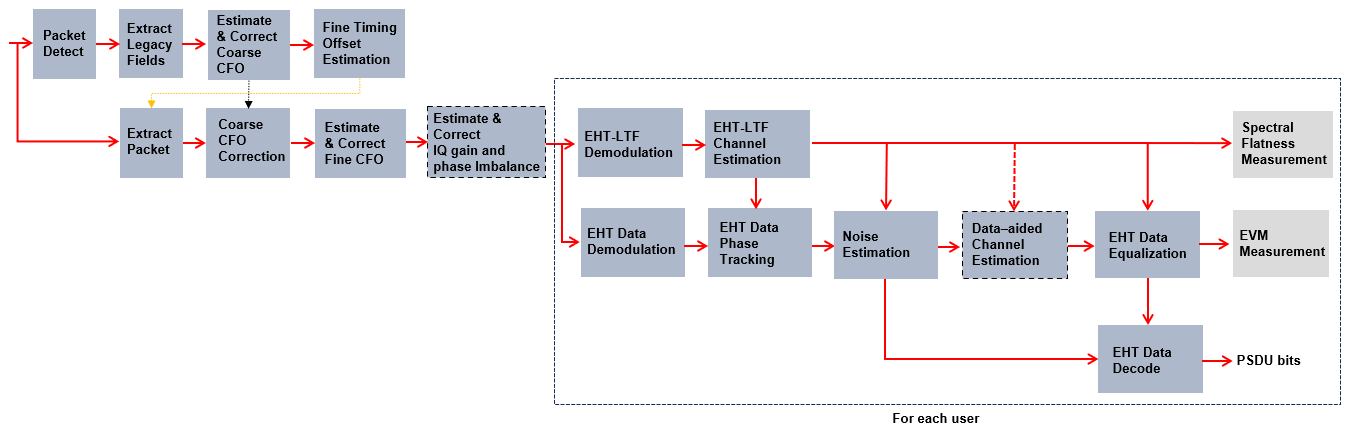This example performs two different EVM measurements.

1. RMS EVM per user per packet, which comprises averaging the EVM over subcarriers, OFDM symbols, and spatial streams.

2. RMS EVM per subcarrier per spatial stream per user for a packet. Because this configuration maps spatial streams directly to antennas, this measurement can help detect frequency-dependent impairments, which tend to affect individual RF chains differently. This measurement averages the EVM over OFDM symbols only.

Get indices for accessing each field within the time-domain packet.

`ind = wlanFieldIndices(cfgEHT);`

Define the minimum detectable length of data, in samples.

`minPktLen = double(ind.LSTF(2)-ind.LSTF(1))+1;`

Detect and process packets within the received waveform by using a `while` loop, which performs these steps.

1. Detect a packet by indexing into `rxWaveform` with the sample offset, `searchOffset`

2. Detect and process the first packet within `rxWaveform`

3. Detect and process the next packet by incrementing the sample index offset

4. Repeat until no further packets are detected

```rxWaveformLength = size(rxWaveform,1); pktLength = double(ind.EHTData(2)); rmsEVM = zeros(numPackets,numUsers); eqSym = cell(1,numUsers); evmPerSC = cell(1,numUsers); decodeSuccess = false(numPackets,numUsers); passSF = false(numPackets,1); pktOffsetStore = zeros(numPackets,1); rng(savedState); % Restore random state pktNum = 0; searchOffset = 0; % Start at first sample (no offset) while (searchOffset+minPktLen)<=rxWaveformLength % Detect packet and determine coarse packet offset pktOffset = wlanPacketDetect(rxWaveform,cfgEHT.ChannelBandwidth,searchOffset); % Packet offset from start of the waveform pktOffset = searchOffset+pktOffset; % Skip packet if L-STF is empty if isempty(pktOffset) || (pktOffset<0) || ... ((pktOffset+ind.LSIG(2))>rxWaveformLength) break; end % Extract L-STF and perform coarse frequency offset correction nonht = rxWaveform(pktOffset+(ind.LSTF(1):ind.LSIG(2)),:); coarsefreqOff = wlanCoarseCFOEstimate(nonht,cfgEHT.ChannelBandwidth); nonht = frequencyOffset(nonht,fs,-coarsefreqOff); % Extract the legacy fields and determine fine packet offset lltfOffset = wlanSymbolTimingEstimate(nonht,cfgEHT.ChannelBandwidth); pktOffset = pktOffset+lltfOffset; % Determine packet offset % If offset is outside the bounds of the waveform, then skip samples % and continue searching within remainder of the waveform if (pktOffset<0) || ((pktOffset+pktLength)>rxWaveformLength) searchOffset = pktOffset+double(ind.LSTF(2))+1; continue; end % Timing synchronization complete; extract the detected packet rxPacket = rxWaveform(pktOffset+(1:pktLength),:); pktNum = pktNum+1; % Apply coarse frequency correction to the extracted packet rxPacket = frequencyOffset(rxPacket,fs,-coarsefreqOff); % Perform fine frequency offset correction on the extracted packet lltf = rxPacket(ind.LLTF(1):ind.LLTF(2),:); % Extract L-LTF fineFreqOff = wlanFineCFOEstimate(lltf,cfgEHT.ChannelBandwidth); rxPacket = frequencyOffset(rxPacket,fs,-fineFreqOff); % Extract EHT-LTF samples, demodulate, and perform channel estimation ehtLTF = rxPacket(ind.EHTLTF(1):ind.EHTLTF(2),:); for i = 1:numUsers ehtLTFDemod = wlanEHTDemodulate(ehtLTF,'EHT-LTF',cfgEHT,i); % Estimate channel [chanEst,pilotEst] = wlanEHTLTFChannelEstimate(ehtLTFDemod,cfgEHT,i); % Measure spectral flatness for non-OFDMA PPDU type if strcmp(ppduType,"Non-OFDMA") [passSF(pktNum),deviation,testsc] = wlanSpectralFlatness(chanEst,'EHT',cfgEHT.ChannelBandwidth); % Plot deviation against limits ehtPlotTxSpectralFlatness(deviation,testsc,pktNum); end % Data demodulate rxData = rxPacket(ind.EHTData(1):ind.EHTData(2),:); demodSym = wlanEHTDemodulate(rxData,'EHT-Data',cfgEHT,i); % Perform pilot phase tracking demodSym = wlanEHTTrackPilotError(demodSym,chanEst,cfgEHT,'EHT-Data',i); % Estimate noise power in EHT fields ofdmInfo = wlanEHTOFDMInfo('EHT-Data',cfgEHT,i); % OFDM parameters nVarEst = ehtNoiseEstimate(demodSym(ofdmInfo.PilotIndices,:,:),pilotEst,cfgEHT,i); % Extract data subcarriers from demodulated symbols and channel % estimate demodDataSym = demodSym(ofdmInfo.DataIndices,:,:); chanEstData = chanEst(ofdmInfo.DataIndices,:,:); % Equalize [eqSym{i},csi] = ehtEqualizeCombine(demodDataSym,chanEstData,nVarEst,cfgEHT,i); % Set up EVM measurements [EVMPerPkt,EVMPerSC] = evmSetup(cfgEHT,i); % Compute RMS EVM over all spatial streams for the packet rmsEVM(pktNum,i) = EVMPerPkt(eqSym{i}); % Compute RMS EVM per subcarrier and spatial stream for the packet evmPerSC{i} = EVMPerSC(eqSym{i}); % Nst-by-1-by-Nss % Recover data field bits rxPSDU = wlanEHTDataBitRecover(eqSym{i},nVarEst,csi,cfgEHT,i); if isequal(rxPSDU,data{i}((1:psduLen(i))+(pktNum-1)*psduLen(i))) decodeSuccess(pktNum,i) = true; end end % Plot equalized constellation and RMS EVM per subcarrier ehtTxEVMConstellationPlots(eqSym,evmPerSC,cfgEHT,pktNum); % Store the offset of each packet within the waveform pktOffsetStore(pktNum) = pktOffset; % Increment waveform offset and search remaining waveform for a packet searchOffset = pktOffset+pktLength+minPktLen; end```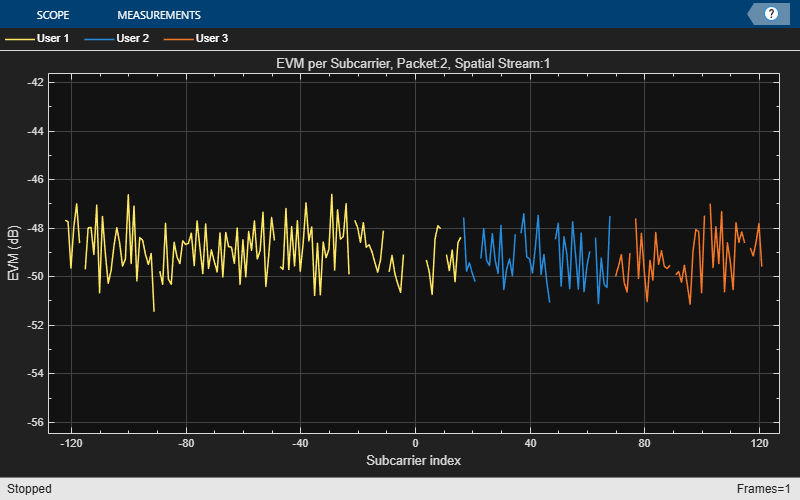Set the unit of EVM to decibel or percentage.

`evmUnit ="Decibel";`

Display tables for decode status and measurement summary.

`ehtMeasurementSummary(cfgEHT,rmsEVM,decodeSuccess,pktOffsetStore,passSF,evmUnit);`
```Decode Status Packet Number Start Index User 1 User 2 User 3 _____________ ___________ _________ _________ _________ 1 74 "Success" "Success" "Success" 2 9074 "Success" "Success" "Success" Measurement Summary Packet Number User 1 EVM (dB) User 2 EVM (dB) User 3 EVM (dB) Packet EVM (dB) _____________ _______________ _______________ _______________ _______________ 1 -49.438 -49.408 -49.607 -49.484 2 -48.78 -49.123 -49.103 -49.001 Average EVM for 3 users: User 1: -49.10dB User 2: -49.26dB User 3: -49.35dB All users: -49.24dB ```

In this section, you measure the spectral mask of the filtered and impaired waveform after HPA modeling. The transmitter spectral mask test  uses a time-gated spectral measurement of the EHT Data field. The example extracts the EHT Data field of each packet from the oversampled waveform by using the start indices of each packet within the baseband waveform. Any delay introduced in the baseband processing chain used to determine the packet indices must be accounted for when gating the EHT Data field within `txWaveform`. Concatenate the extracted EHT Data fields in preparation for measurement.

```startIdx = osf*(ind.EHTData(1)-1)+1; % Upsampled start of EHT Data endIdx = osf*ind.EHTData(2); % Upsampled end of EHT Data delay = grpdelay(firdec,1); % Group delay of downsampling filter idx = zeros(endIdx-startIdx+1,pktNum); for i = 1:pktNum % Start of packet in txWaveform pktOffset = round(osf*pktOffsetStore(i))-delay; % Indices of EHT Data in txWaveform idx(:,i) = (pktOffset+(startIdx:endIdx)); end gatedEHTTData = txWaveform(idx(:),:);```

The 802.11be standard specifies the spectral mask relative to the peak power spectral density (PSD). This plot overlays the required mask with the measured PSD.

```if pktNum>0 ehtSpectralMaskTest(gatedEHTTData,fs,osf); end```
``` Spectral mask passed ```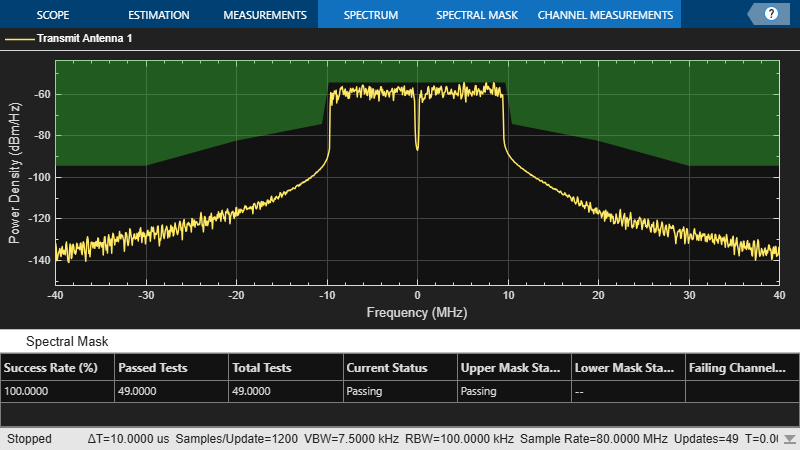### Conclusion and Further Exploration

This example shows how to measure and plot these properties of an IEEE 802.11be waveform.

• Spectral flatness for non-OFDMA PPDU type

• RMS EVM per subcarrier

• Equalized constellation

The HPA model introduces significant in-band distortion and spectral regrowth, which is visible in the EVM results, noisy constellation, and out-of-band emissions in the spectral mask plot. Try increasing the HPA backoff and observe the improved EVM, constellation, and lower out-of-band emissions. The downsampling (to bring the waveform to baseband for processing) stage includes filtering. The filter response affects the spectral flatness measurement. The ripple in the spectral flatness measurement is due to downsampling to baseband. Try using a different filter or changing the stop-band attenuation and observe the impact on the spectral flatness. For meaningful EVM results generate at least 20 EHT MU packets as specified in Section 36.3.19.4.4 of .

### References

1. IEEE P802.11be™/D2.0 Draft Standard for Information technology - Telecommunications and information exchange between systems Local and metropolitan area networks - Specific requirements - Part 11: Wireless LAN Medium Access Control (MAC) and Physical Layer (PHY) Specifications - Amendment 8: Enhancements for extremely high throughput (EHT).

2. IEEE P802.11ax™-2021. Draft Standard for Information technology - Telecommunications and Information Exchange between Systems - Local and Metropolitan Area Networks - Specific Requirements - Part 11: Wireless LAN Medium Access Control (MAC) and Physical Layer (PHY) Specifications - Amendment 1: Enhancements for High Efficiency WLAN.

3. Loc and Cheong. IEEE P802.11 Wireless LANs. TGac Functional Requirements and Evaluation Methodology Rev. 16. 2011-01-19.

4. Perahia, E., and R. Stacey. Next Generation Wireless LANs: 802.11n and 802.11ac. 2nd Edition. United Kingdom: Cambridge University Press, 2013.

5. Archambault, Jerry, and Shravan Surineni. "IEEE 802.11 spectral measurements using vector signal analyzers." RF Design 27.6 (2004): 38-49.